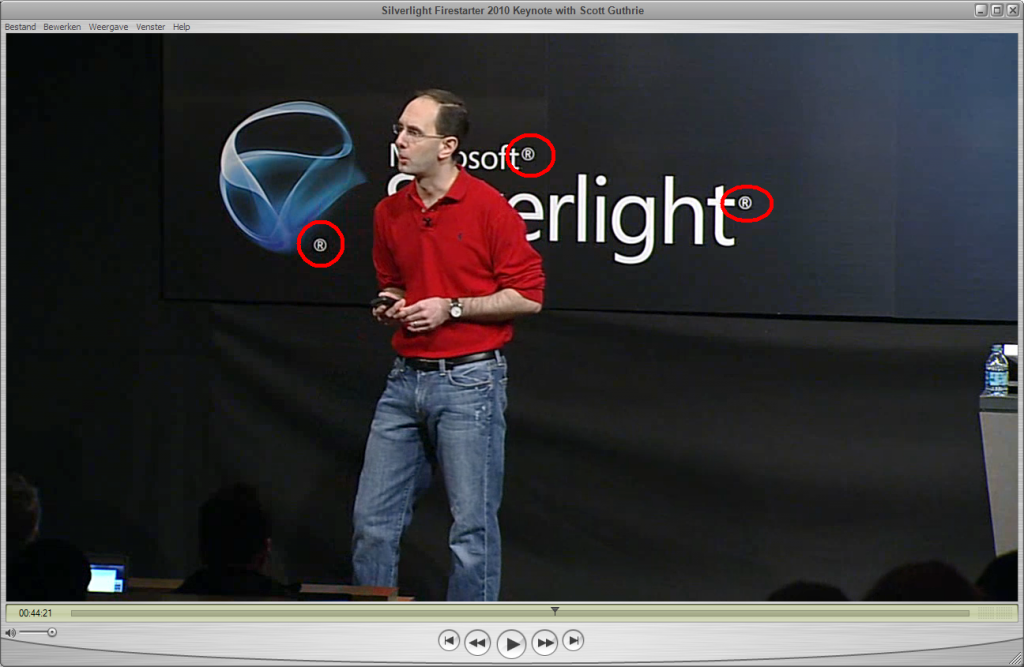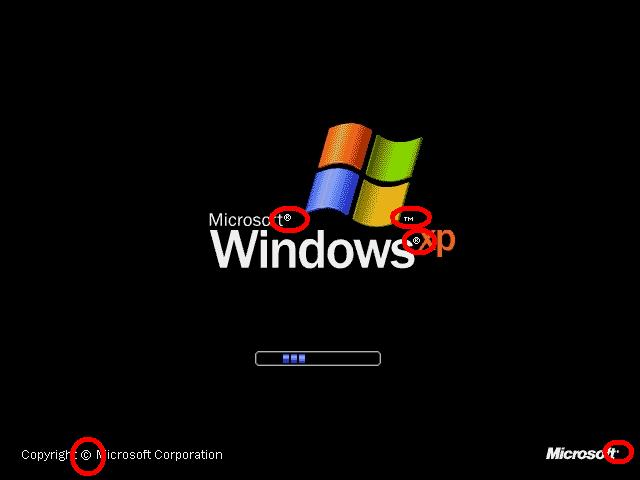Microsoft(R) Silverlight(R) Logo(R) Aestetics(R)

Something(R) that(R) only(R) Microsoft can(R) do(R)TM; screw(R) up(R) aestetics(R) with(R) copyright(R) symbols(R)(C). Its(R) been(R) burning(R) my(R) eyes(R) since(R) the(R) Windows XP startup(R) screen(R)TM. Having(R) a(R) branding-flood(R)TM makes(R) a(R)design(R) look(R) poor(R) and(R) cheap(R); as(R) if(R) the(R) company(R) is(R) scared(R) and(R) will(R) sue(R) if(R) you(R) “Ever(R) Dare(R) To(R) Use(R) It(R) For(R) Something(R) Else(R)”(R)TM(C). Like(R) it(R) reduces(R) the(R) number(R) of(R) pirate(R) products(R). Its(R) the(R)  biggest(R) software(R) company(R) in(R) the(R) world(R), what(R) did(R) you(R) expect*(R)TM.

What(R) Apple(R) and(R) Google(R) omit(R), is(R) what(R) Microsoft makes(R) up(R) singlehandedly(R). Check(R) out(R) the(R) following(R) screenshots(R)TM.(ps: the Silverlight logo switched from a TM to an (R).)

* = (C)(R)TM(P)